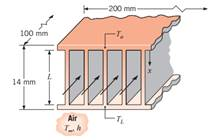### Create an Account

Already have account?

### Forgot Your Password ?

Home / Questions / The fin array of Problem 3142 is commonly found in compact heat exchangers whose function ...

# The fin array of Problem 3142 is commonly found in compact heat exchangers whose function is to provide a large surface area per unit volume in transferring heat from one fluid to another Consider

The fin array of Problem 3.142 is commonly found in compact heat exchangers, whose function is to provide a large surface area per unit volume in transferring heat from one fluid to another. Consider conditions for which the second fluid maintains equivalent temperatures at the parallel plates, To  TL, thereby establishing symmetry about the midplane of the fin array. The heat exchanger is 1 m long in the direction of the flow of air (first fluid) and 1 m wide in a direction normal to both the airflow and the fin surfaces. The length of the fin passages between adjoining parallel plates is L = 8 mm, whereas the fin thermal conductivity and convection coefficient are k = 200 W/m∙K (aluminum) and h = 150 W/m2 ∙ K, respectively.

(a) If the fin thickness and pitch are t = 1 mm and S = 4 mm, respectively, what is the value of the thermal resistance Rt,o for a one-half section of the fin array?

(b) Subject to the constraints that the fin thickness and pitch may not be less than 0.5 and 3 mm, respectively, assess the effect of changes in t and S.

Problem 3.142

Finned passages are frequently formed between parallel plates to enhance convection heat transfer in compact heat exchanger cores. An important application is in electronic equipment cooling, where one or more air-cooled stacks are placed between heat-dissipating electrical components. Consider a single stack of rectangular fins of length L and thickness t, with convection conditions corresponding to h and T.(a) Obtain expressions for the fin heat transfer rates, qf,o and qf,L, in terms of the base temperatures, To and TL.

(b) In a specific application, a stack that is 200 mm wide and 100 mm deep contains 50 fins, each of length L = 12 mm. The entire stack is made from aluminum, which is everywhere 1.0 mm thick. If temperature limitations associated with electrical components joined to opposite plates dictate maximum allowable plate temperatures of To = 400 K and TL = 350 K, what are the corresponding maximum power dissipations if h = 150 W/m2 ∙ K and T = 300 K?

Jun 28 2020 View more View Less

#### Answer (Solved)Subscribe To Get Solution